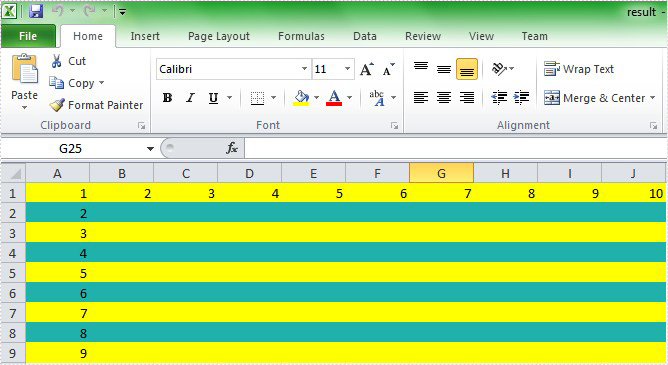## Conditional Formatting (10)

Thursday, 19 April 2018 09:14

### Highlight Below and Above Average Values in Excel in C#

Written by support iceblue

This article demonstrates the steps to highlight below and above average values with conditional formatting in Excel using Spire.XLS.

Below screenshot shows the sample file we used for demonstration: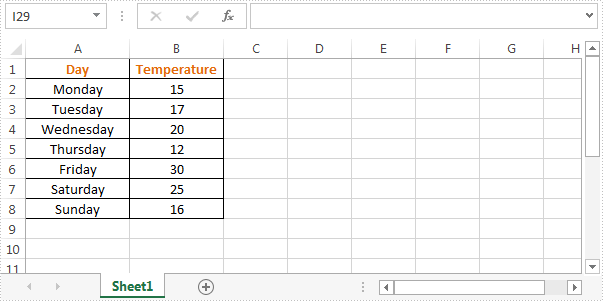Detail steps:

Step 1: Instantiate a Workbook object and load the Excel file.

```Workbook workbook = new Workbook();
```

Step 2: Get the first worksheet.

```Worksheet sheet = workbook.Worksheets;
```

Step 3: Highlight cells below average values with SkyBlue color.

```//Add conditional format
//Set the cell range to apply the formatting
//Highlight cells below average values
cf1.BackColor = Color.SkyBlue;
```

Step 4: Highlight cells above average values with Orange color.

```//Add conditional format
//Set the cell range to apply the formatting
//Highlight cells above average values
cf2.BackColor = Color.Orange;
```

Step 5: Save the file.

```workbook.SaveToFile("AverageCondition.xlsx", ExcelVersion.Version2013);
```

Screenshot: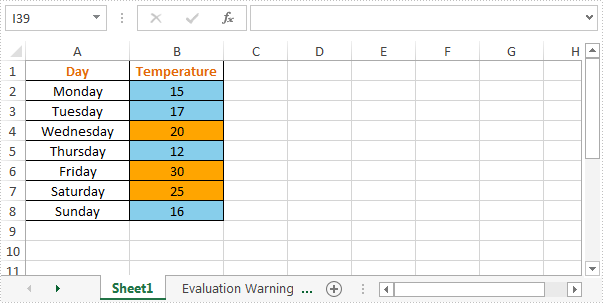Full code:

```using System.Drawing;
using Spire.Xls;
using Spire.Xls.Core;

namespace Average_Condition
{
class Program
{
static void Main(string[] args)
{
//Instantiate a Workbook object
Workbook workbook = new Workbook();

//Get the first worksheet
Worksheet sheet = workbook.Worksheets;

//Set the cell range to apply the formatting
//Highlight cells below average values
cf1.BackColor = Color.SkyBlue;

//Set the cell range to apply the formatting
//Highlight cells above average values
cf2.BackColor = Color.Orange;

//Save the file
workbook.SaveToFile("AverageCondition.xlsx", ExcelVersion.Version2013);
}
}
}
```
Monday, 25 December 2017 08:38

### Highlight Top and Bottom Ranked Values in Excel Using C#

Written by support iceblue

One of the best uses of conditional formatting is to quickly highlight top or bottom ranked values in a large set of data. In this article, we’re going to show you how to highlight top and bottom ranked values in an Excel worksheet using Spire.XLS and conditional formatting.

The input.xlsx file we used for demonstration: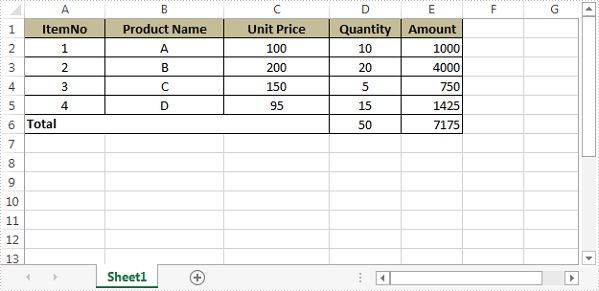Detail steps:

Step 1: Initialize an object of Workbook class and load the Excel file.

```Workbook workbook = new Workbook();
```

Step 2: Get the first worksheet.

```Worksheet sheet = workbook.Worksheets;
```

Step 3: Apply conditional formatting to range "C2:C5" to highlight the top 2 ranked values.

```ConditionalFormatWrapper format1 = sheet.Range["C2:C5"].ConditionalFormats.AddCondition();
format1.FormatType = ConditionalFormatType.TopBottom;
format1.TopBottom.Type = TopBottomType.Top;
format1.TopBottom.Rank = 2;
format1.BackColor = Color.Red;
```

Step 4: Apply conditional formatting to range "D2:D5" to highlight the bottom 2 ranked values.

```ConditionalFormatWrapper format2 = sheet.Range["D2:D5"].ConditionalFormats.AddCondition();
format2.FormatType = ConditionalFormatType.TopBottom;
format2.TopBottom.Type = TopBottomType.Bottom;
format2.TopBottom.Rank = 2;
format2.BackColor = Color.ForestGreen;
```

Step 5: Save the file.

```workbook.SaveToFile("TopBottomValues.xlsx", ExcelVersion.Version2013);
```

Screenshot: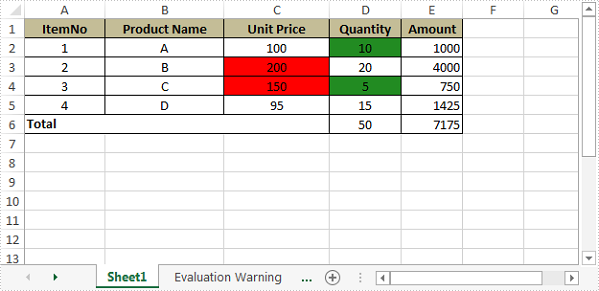Full code:

```//Initialize an object of Workbook class
Workbook workbook = new Workbook();

//Get the first worksheet
Worksheet sheet = workbook.Worksheets;

//Apply conditional formatting to range “C2:C5” to highlight the top 2 values
format1.FormatType = ConditionalFormatType.TopBottom;
format1.TopBottom.Type = TopBottomType.Top;
format1.TopBottom.Rank = 2;
format1.BackColor = Color.Red;

//Apply conditional formatting to range “D2:D5” to highlight the bottom 2 values
format2.FormatType = ConditionalFormatType.TopBottom;
format2.TopBottom.Type = TopBottomType.Bottom;
format2.TopBottom.Rank = 2;
format2.BackColor = Color.ForestGreen;

//Save the file
workbook.SaveToFile("TopBottomValues.xlsx", ExcelVersion.Version2013);
```
Tuesday, 12 December 2017 09:38

### Highlight Duplicate and Unique Values in Excel Using C#

Written by support iceblue

Using Excel conditional formatting, we can quickly find and highlight the duplicate and unique values in a selected cell range. In this article, we’re going to show you how to programmatically highlight duplicate and unique values with different colors using Spire.XLS and conditional formatting.

Detail steps:

Step 1: Initialize an object of Workbook class and Load the Excel file.

```Workbook workbook = new Workbook();
```

Step 2: Get the first worksheet.

```Worksheet sheet = workbook.Worksheets;
```

Step 3: Use conditional formatting to highlight duplicate values in range "A2:A10" with IndianRed color.

```ConditionalFormatWrapper format1 = sheet.Range["A2:A10"].ConditionalFormats.AddCondition();
format1.FormatType = ConditionalFormatType.DuplicateValues;
format1.BackColor = Color.IndianRed;
```

Step 4: Use conditional formatting to highlight unique values in range "A2:A10" with Yellow color.

```ConditionalFormatWrapper format2 = sheet.Range["A2:A10"].ConditionalFormats.AddCondition();
format2.FormatType = ConditionalFormatType.UniqueValues;
format2.BackColor = Color.Yellow;
```

Step 5: Save the file.

```workbook.SaveToFile("HighlightDuplicates.xlsx", ExcelVersion.Version2013);
```

Screenshot: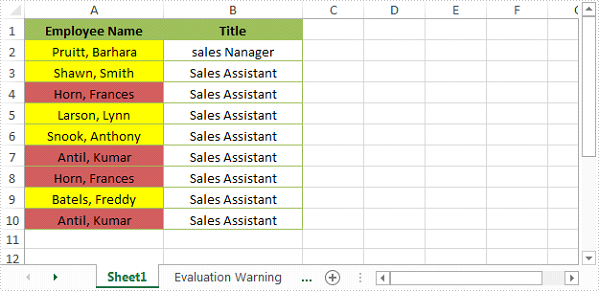Full code:

```//Load the Excel file
Workbook workbook = new Workbook();

//Get the first worksheet
Worksheet sheet = workbook.Worksheets;

//Use conditional formatting to highlight duplicate values in range "A2:A10" with IndianRed color
format1.FormatType = ConditionalFormatType.DuplicateValues;
format1.BackColor = Color.IndianRed;

//Use conditional formatting to highlight unique values in range "A2:A10" with Yellow color
format2.FormatType = ConditionalFormatType.UniqueValues;
format2.BackColor = Color.Yellow;

//Save the file
workbook.SaveToFile("HighlightDuplicates.xlsx", ExcelVersion.Version2013);
```
Friday, 08 December 2017 02:13

### Conditionally Format Dates in Excel with C#

Written by jie zou

Microsoft Excel provides 10 date options, ranging from yesterday to next month (see below image) to format selected cells based on the current date. Spire.XLS supports all of these options, in this article, we’re going to show you how to conditionally format dates in Excel using Spire.XLS. If you want to highlight cells based on a date in another cell, or create rules for other dates (i.e., more than a month from the current date), you will have to create your own conditional formatting rule based on a formula.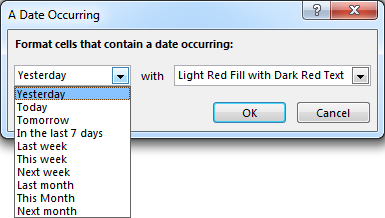Detail steps:

Step 1: Initialize an object of Workbook class and load the Excel file.

```Workbook workbook = new Workbook();
```

Step 2: Get the first worksheet.

```Worksheet sheet = workbook.Worksheets;
```

Step 3: Add a condition to the used range in the worksheet.

```ConditionalFormatWrapper conditionalFormat = sheet.AllocatedRange.ConditionalFormats.AddCondition();
```

Step 4: Specify the format type of the condition as time period and set the time period as last 7 days.

```conditionalFormat.FormatType = ConditionalFormatType.TimePeriod;
conditionalFormat.SetTimePeriod(TimePeriodType.Last7Days);
```

Step 5:Set the highlight color.

```conditionalFormat.BackColor = Color.Orange;
```

Step 6:Save the file.

```workbook.SaveToFile("ConditionallyFormatDates.xlsx", ExcelVersion.Version2013);
```

Screenshot::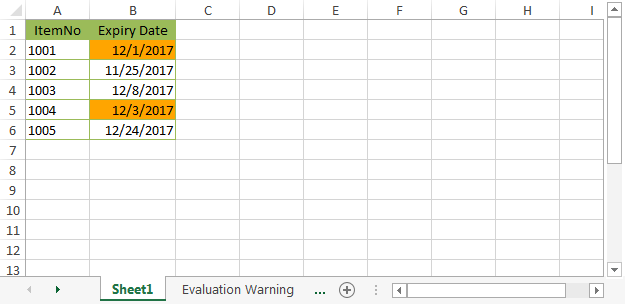Full Code:

[C#]
```//Initialize an object of Workbook class
Workbook workbook = new Workbook();

//Get the first worksheet
Worksheet sheet = workbook.Worksheets;

//Highlight cells that contain a date occurring in the last 7 days
conditionalFormat.FormatType = ConditionalFormatType.TimePeriod;
conditionalFormat.SetTimePeriod(TimePeriodType.Last7Days);
conditionalFormat.BackColor = Color.Orange;

//Save the file
workbook.SaveToFile("ConditionallyFormatDates.xlsx", ExcelVersion.Version2013);
```
Thursday, 07 September 2017 03:17

### Remove conditional format from Excel in C#

Written by support iceblue

With the help of Spire.XLS, we can set the conditional format the Excel cell in C# and VB.NET. We can also use Spire.XLS to remove the conditional format from a specific cell or the entire Excel worksheet. This article will demonstrate how to remove conditional format from Excel in C#.

Firstly, view the original Excel worksheet with conditional formats: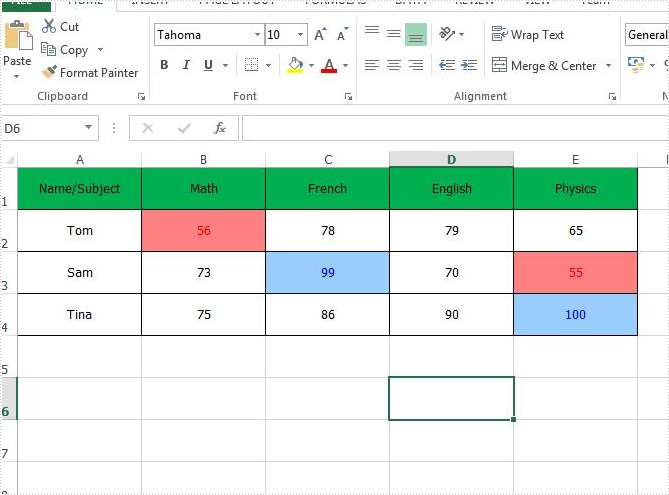Step 1: Create an instance of Excel workbook and load the document from file.

```Workbook workbook = new Workbook();
```

Step 2: Get the first worksheet from the workbook.

```Worksheet sheet = workbook.Worksheets;
```

Step 3: Remove the conditional formats from the specific Excel Cell B2.

```sheet.Range["B2"].ConditionalFormats.Remove();
```

Step 4: Remove all the conditional formats from the whole Excel worksheet.

```sheet.AllocatedRange.ConditionalFormats.Remove();
```

Step 5: Save the document to file.

```workbook.SaveToFile("Result.xlsx", ExcelVersion.Version2010);
```

Remove the conditional format from a special Excel range B2: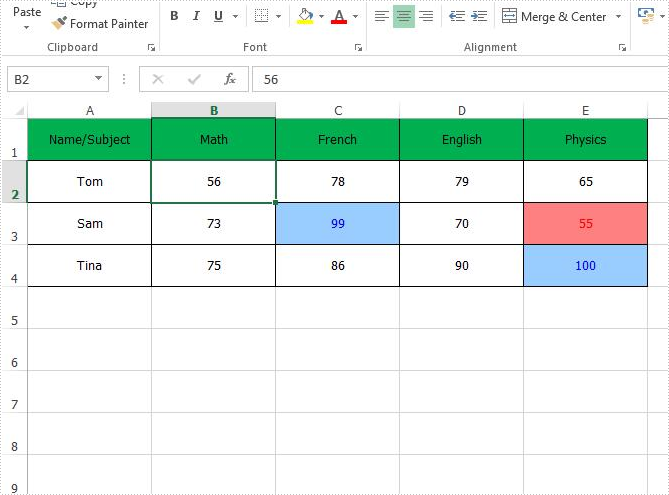Remove all the conditional formats from the entire Excel worksheet: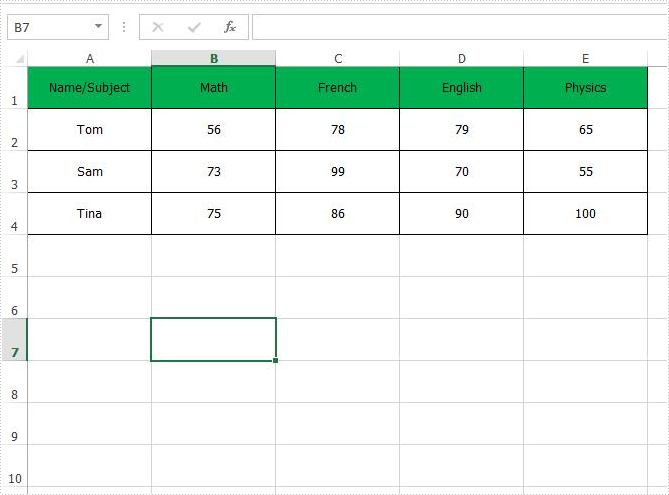Full codes of how to remove the conditional formats from Excel worksheet:

```Workbook workbook = new Workbook();

Worksheet sheet = workbook.Worksheets;

//sheet.Range["B2"].ConditionalFormats.Remove();

sheet.AllocatedRange.ConditionalFormats.Remove();

workbook.SaveToFile("Result.xlsx", ExcelVersion.Version2010);
```
Wednesday, 11 May 2016 07:40

### How to create a formula to apply conditional formatting in Excel in C#

Written by support iceblue

We always use conditional formatting to highlight the cells with certain color from the whole data in the Excel worksheet. Spire.XLS also supports to create a formula to apply conditional formatting in Excel in C#. This article will show you how to apply a conditional formatting rule.

View the steps of Microsoft Excel set the conditional formatting.

Step 1: Choose the column B and then click "New Rule" under "Conditional Formatting"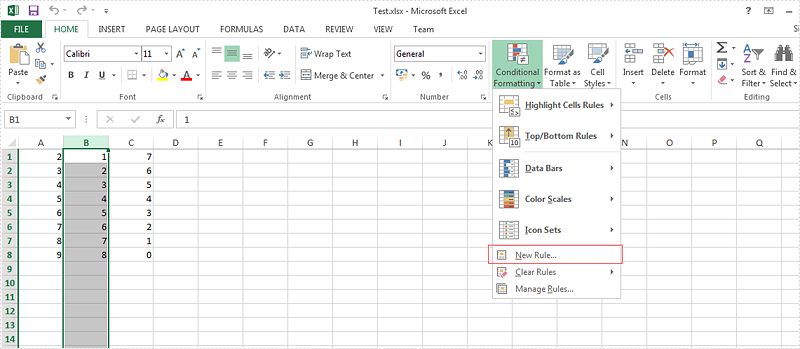Step 2: Select the rule type, enter the rule by adding the formula and then add the highlight color for the format.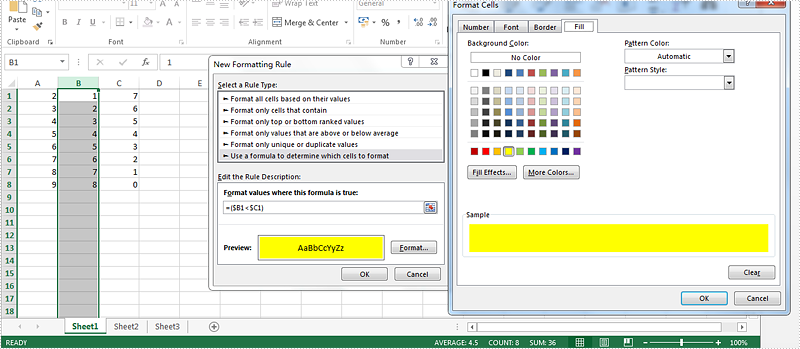Here comes to the steps of how to set the conditional formatting rule by Spire.XLS in C#.

Step 1: Create a new excel workbook and load the document from file.

```Workbook wb = new Workbook();
```

Step 2: Get the first worksheet and the second column from the workbook.

```Worksheet sheet = wb.Worksheets;
CellRange range = sheet.Columns;
```

Step 3: Set the conditional formatting formula and apply the rule to the chosen cell range.

```ConditionalFormatWrapper conditional = range.ConditionalFormats.AddCondition();
conditional.FormatType = ConditionalFormatType.Formula;
conditional.FirstFormula = "=(\$B1<\$C1)";
conditional.BackKnownColor = ExcelColors.Yellow;
```

Step 4: Save the document to file.

```wb.SaveToFile("result.xlsx", ExcelVersion.Version2010);
```

Effective screenshot:Full codes:

```static void Main(string[] args)
{
Workbook wb = new Workbook();

Worksheet sheet = wb.Worksheets;
CellRange range = sheet.Columns;

conditional.FormatType = ConditionalFormatType.Formula;
conditional.FirstFormula = "=(\$B1<\$C1)";
conditional.BackKnownColor = ExcelColors.Yellow;

wb.SaveToFile("result.xlsx", ExcelVersion.Version2010);

}
```
Monday, 15 February 2016 08:26

### How to set the traffic lights icons in C# by Spire.XLS

Written by support iceblue

By using Spire.XLS, developers can easily set the IconSetType of conditional formatting. This article will demonstrate how to set the traffic lights icons in C# with the help of Spire.XLS.

Here comes to the code snippets:

Step 1: Create a new excel document instance and get the first worksheet.

```Workbook wb = new Workbook();
Worksheet sheet = book.Worksheets;
```

Step 2: Add some data to the Excel sheet cell range and set the format for them.

```sheet.Range["A1"].Text = "Traffic Lights";
sheet.Range["A2"].NumberValue = 0.95;
sheet.Range["A2"].NumberFormat = "0%";
sheet.Range["A3"].NumberValue = 0.5;
sheet.Range["A3"].NumberFormat = "0%";
sheet.Range["A4"].NumberValue = 0.1;
sheet.Range["A4"].NumberFormat = "0%";
sheet.Range["A5"].NumberValue = 0.9;
sheet.Range["A5"].NumberFormat = "0%";
sheet.Range["A6"].NumberValue = 0.7;
sheet.Range["A6"].NumberFormat = "0%";
sheet.Range["A7"].NumberValue = 0.6;
sheet.Range["A7"].NumberFormat = "0%";
```

Step 3: Set the height of row and width of column for Excel cell range.

```sheet.AllocatedRange.RowHeight = 20;
sheet.AllocatedRange.ColumnWidth = 25;
```

Step 4: Add a conditional formatting of cell range and set its type to CellValue.

```ConditionalFormatWrapper format1 = sheet.Range.ConditionalFormats.AddCondition();
format1.FormatType = ConditionalFormatType.CellValue;
format1.FirstFormula = "300";
format1.Operator = ComparisonOperatorType.Less;
format1.FontColor = Color.Black;
format1.BackColor = Color.LightSkyBlue;
```

Step 5: Add a conditional formatting of cell range and set its type to IconSet.

```ConditionalFormatWrapper format = sheet.AllocatedRange.ConditionalFormats.AddCondition();
format.FormatType = ConditionalFormatType.IconSet;
format.IconSet.IconSetType = IconSetType.ThreeTrafficLights1;
```

Step 6: Save the document to file.

```wb.SaveToFile("Light.xlsx", ExcelVersion.Version2010);
```

Effective screenshots of the traffic lights icons set by Spire.XLS.Monday, 31 August 2015 01:31

### How to format cells with borders in conditional formatting

Written by support iceblue

Using conditional formatting in Excel, we could highlight interesting cells, emphasize unusual values and visualize data with Data Bars, Color Scales and Icon Sets based on criteria. In the two articles Alternate Row Colors in Excel with Conditional Formatting and Apply Conditional Formatting to a Data Range, we have introduce the method to set fill, font, data bars, color scales and icon sets in conditional formatting using Spire.XLS. This article is going to introduce the method to format cells with borders in conditional formatting.

Step 1: Create a new workbook and add sample data.

```            Workbook workbook = new Workbook();
Worksheet sheet = workbook.Worksheets;

sheet.Range["A1"].Value = "Name/Subject";
sheet.Range["A2"].Value = "Tom";
sheet.Range["A3"].Value = "Sam";
sheet.Range["A4"].Value = "Tina";
sheet.Range["A5"].Value = "Nancy";
sheet.Range["A6"].Value = "James";
sheet.Range["A7"].Value = "Victor";
sheet.Range["B1"].Value = "Math";
sheet.Range["C1"].Value = "French";
sheet.Range["D1"].Value = "English";
sheet.Range["E1"].Value = "Physics";
sheet.Range["B2"].NumberValue = 56;
sheet.Range["B3"].NumberValue = 73;
sheet.Range["B4"].NumberValue = 75;
sheet.Range["B5"].NumberValue = 89;
sheet.Range["B6"].NumberValue = 65;
sheet.Range["B7"].NumberValue = 90;
sheet.Range["C2"].NumberValue = 78;
sheet.Range["C3"].NumberValue = 99;
sheet.Range["C4"].NumberValue = 86;
sheet.Range["C5"].NumberValue = 45;
sheet.Range["C6"].NumberValue = 70;
sheet.Range["C7"].NumberValue = 83;
sheet.Range["D2"].NumberValue = 79;
sheet.Range["D3"].NumberValue = 70;
sheet.Range["D4"].NumberValue = 90;
sheet.Range["D5"].NumberValue = 87;
sheet.Range["D6"].NumberValue = 56;
sheet.Range["D7"].NumberValue = 78;
sheet.Range["E2"].NumberValue = 65;
sheet.Range["E3"].NumberValue = 55;
sheet.Range["E4"].NumberValue = 100;
sheet.Range["E5"].NumberValue = 85;
sheet.Range["E6"].NumberValue = 60;
sheet.Range["E7"].NumberValue = 75;

sheet.AllocatedRange.RowHeight = 17;
sheet.AllocatedRange.ColumnWidth = 17;
sheet.AllocatedRange.VerticalAlignment = VerticalAlignType.Center;
sheet.AllocatedRange.HorizontalAlignment = HorizontalAlignType.Center;
```

Step 2: Set the formatting rule using formula. Here the rule is the number values less than 60.

```            ConditionalFormatWrapper format1 = sheet.Range["B2:E7"].ConditionalFormats.AddCondition();
format1.FirstFormula = "60";
format1.Operator = ComparisonOperatorType.Less;
```

Step 3: Set border colors and styles for cells that match the condition.

```            format1.LeftBorderColor = Color.Red;
format1.RightBorderColor = Color.DarkBlue;
format1.TopBorderColor = Color.DeepSkyBlue;
format1.BottomBorderColor = Color.DeepSkyBlue;

format1.LeftBorderStyle = LineStyleType.Medium;
format1.RightBorderStyle = LineStyleType.Thick;
format1.TopBorderStyle = LineStyleType.Double;
format1.BottomBorderStyle = LineStyleType.Double;
```

Step 4: Save the document and launch to see effects.

```            workbook.SaveToFile("sample.xlsx", ExcelVersion.Version2010);
System.Diagnostics.Process.Start("sample.xlsx");
```

Effects: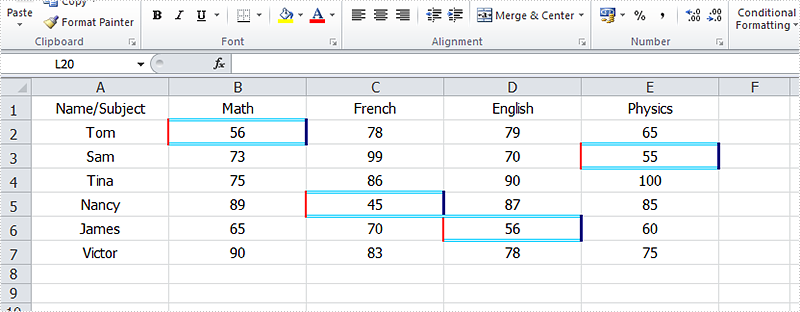Full Codes:

```using System;
using System.Collections.Generic;
using System.Linq;
using System.Text;
using Spire.Xls;
using System.Drawing;

namespace Test
{
class Program
{
static void Main(string[] args)
{
Workbook workbook = new Workbook();
Worksheet sheet = workbook.Worksheets;

sheet.Range["A1"].Value = "Name/Subject";
sheet.Range["A2"].Value = "Tom";
sheet.Range["A3"].Value = "Sam";
sheet.Range["A4"].Value = "Tina";
sheet.Range["A5"].Value = "Nancy";
sheet.Range["A6"].Value = "James";
sheet.Range["A7"].Value = "Victor";
sheet.Range["B1"].Value = "Math";
sheet.Range["C1"].Value = "French";
sheet.Range["D1"].Value = "English";
sheet.Range["E1"].Value = "Physics";
sheet.Range["B2"].NumberValue = 56;
sheet.Range["B3"].NumberValue = 73;
sheet.Range["B4"].NumberValue = 75;
sheet.Range["B5"].NumberValue = 89;
sheet.Range["B6"].NumberValue = 65;
sheet.Range["B7"].NumberValue = 90;
sheet.Range["C2"].NumberValue = 78;
sheet.Range["C3"].NumberValue = 99;
sheet.Range["C4"].NumberValue = 86;
sheet.Range["C5"].NumberValue = 45;
sheet.Range["C6"].NumberValue = 70;
sheet.Range["C7"].NumberValue = 83;
sheet.Range["D2"].NumberValue = 79;
sheet.Range["D3"].NumberValue = 70;
sheet.Range["D4"].NumberValue = 90;
sheet.Range["D5"].NumberValue = 87;
sheet.Range["D6"].NumberValue = 56;
sheet.Range["D7"].NumberValue = 78;
sheet.Range["E2"].NumberValue = 65;
sheet.Range["E3"].NumberValue = 55;
sheet.Range["E4"].NumberValue = 100;
sheet.Range["E5"].NumberValue = 85;
sheet.Range["E6"].NumberValue = 60;
sheet.Range["E7"].NumberValue = 75;

sheet.AllocatedRange.RowHeight = 17;
sheet.AllocatedRange.ColumnWidth = 17;
sheet.AllocatedRange.VerticalAlignment = VerticalAlignType.Center;
sheet.AllocatedRange.HorizontalAlignment = HorizontalAlignType.Center;

format1.FirstFormula = "60";
format1.Operator = ComparisonOperatorType.Less;

format1.LeftBorderColor = Color.Red;
format1.RightBorderColor = Color.DarkBlue;
format1.TopBorderColor = Color.DeepSkyBlue;
format1.BottomBorderColor = Color.DeepSkyBlue;

format1.LeftBorderStyle = LineStyleType.Medium;
format1.RightBorderStyle = LineStyleType.Thick;
format1.TopBorderStyle = LineStyleType.Double;
format1.BottomBorderStyle = LineStyleType.Double;

workbook.SaveToFile("sample.xlsx", ExcelVersion.Version2010);
System.Diagnostics.Process.Start("sample.xlsx");

}
}
}
```
Friday, 13 March 2015 07:22

### How to Apply Conditional Formatting to a Data Range in C#

Written by support iceblue

Conditional formatting in Microsoft Excel has a number of presets that enables users to apply predefined formatting such as colors, icons and data bars, to a range of cells based on the value of the cell or the value of a formula. Conditional formatting usually reveals the data trends or highlights the data that meets one or more formulas.

In this article, I made an example to explain how these conditional formatting types can be achieved programmatically using Spire.XLS in C#. First of all, let's see the worksheet that contains a group of data in selected range as below, we’d like see which cells’ value is bigger than 800. In order to quickly figure out similar things like this, we can create a conditional formatting rule by formula: “If the value is bigger than 800, color the cell with Red” to highlight the qualified cells.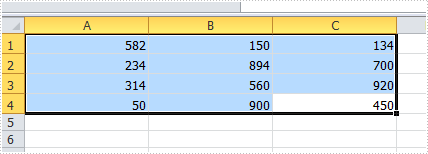#### Code Snippet for Creating Conditional Formatting Rules:

Step 1: Create a worksheet and insert data to cell range from A1 to C4.

```Workbook workbook = new Workbook();
Worksheet sheet = workbook.Worksheets;

sheet.Range["A1"].NumberValue = 582;
sheet.Range["A2"].NumberValue = 234;
sheet.Range["A3"].NumberValue = 314;
sheet.Range["A4"].NumberValue = 50;
sheet.Range["B1"].NumberValue = 150;
sheet.Range["B2"].NumberValue = 894;
sheet.Range["B3"].NumberValue = 560;
sheet.Range["B4"].NumberValue = 900;
sheet.Range["C1"].NumberValue = 134;
sheet.Range["C2"].NumberValue = 700;
sheet.Range["C3"].NumberValue = 920;
sheet.Range["C4"].NumberValue = 450;
sheet.AllocatedRange.RowHeight = 15;
sheet.AllocatedRange.ColumnWidth = 17;
```

Step 2: Create one conditional formatting rule to highlight cells that are greater than 800, and another rule that enables to highlight cells lesser than 300. In our program, the rule is represented by formula. As is shown in the code below, we firstly initialize a new instance of ConditionalFormatWrapper class and apply the format1 to selected cell range. Then define the format1 by setting the related properties. The FirstFormula and Operater property allow us to find out which cells are greater than 800; the Color property enables to color the cells we find. Repeat this method to create format2 to get the cells under 300 highlighted.

```ConditionalFormatWrapper format1 = sheet.AllocatedRange.ConditionalFormats.AddCondition();
format1.FormatType = ConditionalFormatType.CellValue;
format1.FirstFormula = "800";
format1.Operator = ComparisonOperatorType.Greater;
format1.FontColor = Color.Red;
format1.BackColor = Color.LightSalmon;

format2.FormatType = ConditionalFormatType.CellValue;
format2.FirstFormula = "300";
format2.Operator = ComparisonOperatorType.Less;
format2.FontColor = Color.Green;
format2.BackColor = Color.LightBlue;
```

Step 3: Save and launch the file

```workbook.SaveToFile("sample.xlsx", ExcelVersion.Version2010);
System.Diagnostics.Process.Start("sample.xlsx");
```

Result:

The cells with value bigger than 800 and smaller than 300, have been highlighted with defined text color and background color.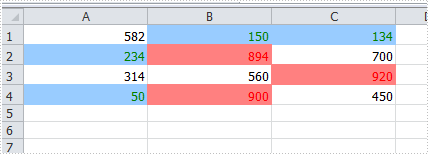#### Apply the Other Three Conditional Formatting Types:

Spire.XLS also supports applying some other conditional formatting types which were predefined in MS Excel. Use the following code snippets to get more formatting effects.

Apply Data Bars:

```ConditionalFormatWrapper format = sheet.AllocatedRange.ConditionalFormats.AddCondition();
format.FormatType = ConditionalFormatType.DataBar;
```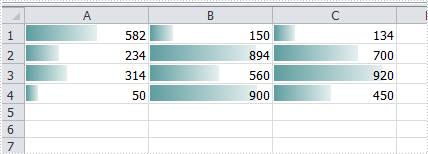Apply Icon Sets:

```ConditionalFormatWrapper format = sheet.AllocatedRange.ConditionalFormats.AddCondition();
format.FormatType = ConditionalFormatType.IconSet;
```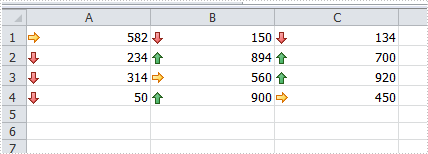Apply Color Scales:

```ConditionalFormatWrapper format = sheet.AllocatedRange.ConditionalFormats.AddCondition();
format.FormatType = ConditionalFormatType.ColorScale;
```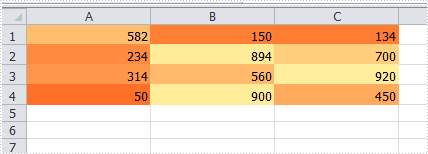Full Code:

```Workbook workbook = new Workbook();
Worksheet sheet = workbook.Worksheets;
sheet.Range["A1"].NumberValue = 582;
sheet.Range["A2"].NumberValue = 234;
sheet.Range["A3"].NumberValue = 314;
sheet.Range["A4"].NumberValue = 50;
sheet.Range["B1"].NumberValue = 150;
sheet.Range["B2"].NumberValue = 894;
sheet.Range["B3"].NumberValue = 560;
sheet.Range["B4"].NumberValue = 900;
sheet.Range["C1"].NumberValue = 134;
sheet.Range["C2"].NumberValue = 700;
sheet.Range["C3"].NumberValue = 920;
sheet.Range["C4"].NumberValue = 450;
sheet.AllocatedRange.RowHeight = 15;
sheet.AllocatedRange.ColumnWidth = 17;

//create conditional formatting rule
format1.FormatType = ConditionalFormatType.CellValue;
format1.FirstFormula = "800";
format1.Operator = ComparisonOperatorType.Greater;
format1.FontColor = Color.Red;
format1.BackColor = Color.LightSalmon;

format2.FormatType = ConditionalFormatType.CellValue;
format2.FirstFormula = "300";
format2.Operator = ComparisonOperatorType.Less;
format2.FontColor = Color.Green;
format2.BackColor = Color.LightBlue;

//format.FormatType = ConditionalFormatType.DataBar;

//format.FormatType = ConditionalFormatType.IconSet;

//format.FormatType = ConditionalFormatType.ColorScale;

workbook.SaveToFile("sample.xlsx", ExcelVersion.Version2010);
System.Diagnostics.Process.Start("sample.xlsx");
```
Monday, 02 December 2013 08:20

### How to Alternate Row Colors in Excel with Conditional Formatting

Like word, data stored in Excel has format. Sometimes, we need to change the format of the data. Spire.XLS, an easy but powerful Excel .NET component can meet your need both in data dealing and format settings.

In this article, we will introduce you how to alternate row colors with conditional formatting.

Step 1: Select the range that you want to format

```CellRange dataRange = sheet.AllocatedRange;
```

Step 2: Set conditional formation

Call the method AddCondition to add a new conditional formatting instance format1.

• FirstFormula : It determines which cells to format.
• FormatType : Set conditional formatting type
• BackColor : Set as LightSeaGreen.
• format1 sets the backcolor of the even rows as LightSeaGreen.
```ConditionalFormatWrapper format1 = dataRange.ConditionalFormats.AddCondition();
format1.FirstFormula = "=MOD(ROW(),2)=0";
format1.FormatType = ConditionalFormatType.Formula;
format1.BackColor = Color.LightSeaGreen;
```

format2 sets the backcolor of the odd rows as Yellow.

Full code:

```static void Main(string[] args)
{
Workbook workbook = new Workbook();
Worksheet sheet = workbook.Worksheets;
CellRange dataRange = sheet.AllocatedRange;

format1.FirstFormula = "=MOD(ROW(),2)=0";
format1.FormatType = ConditionalFormatType.Formula;
format1.BackColor = Color.LightSeaGreen;